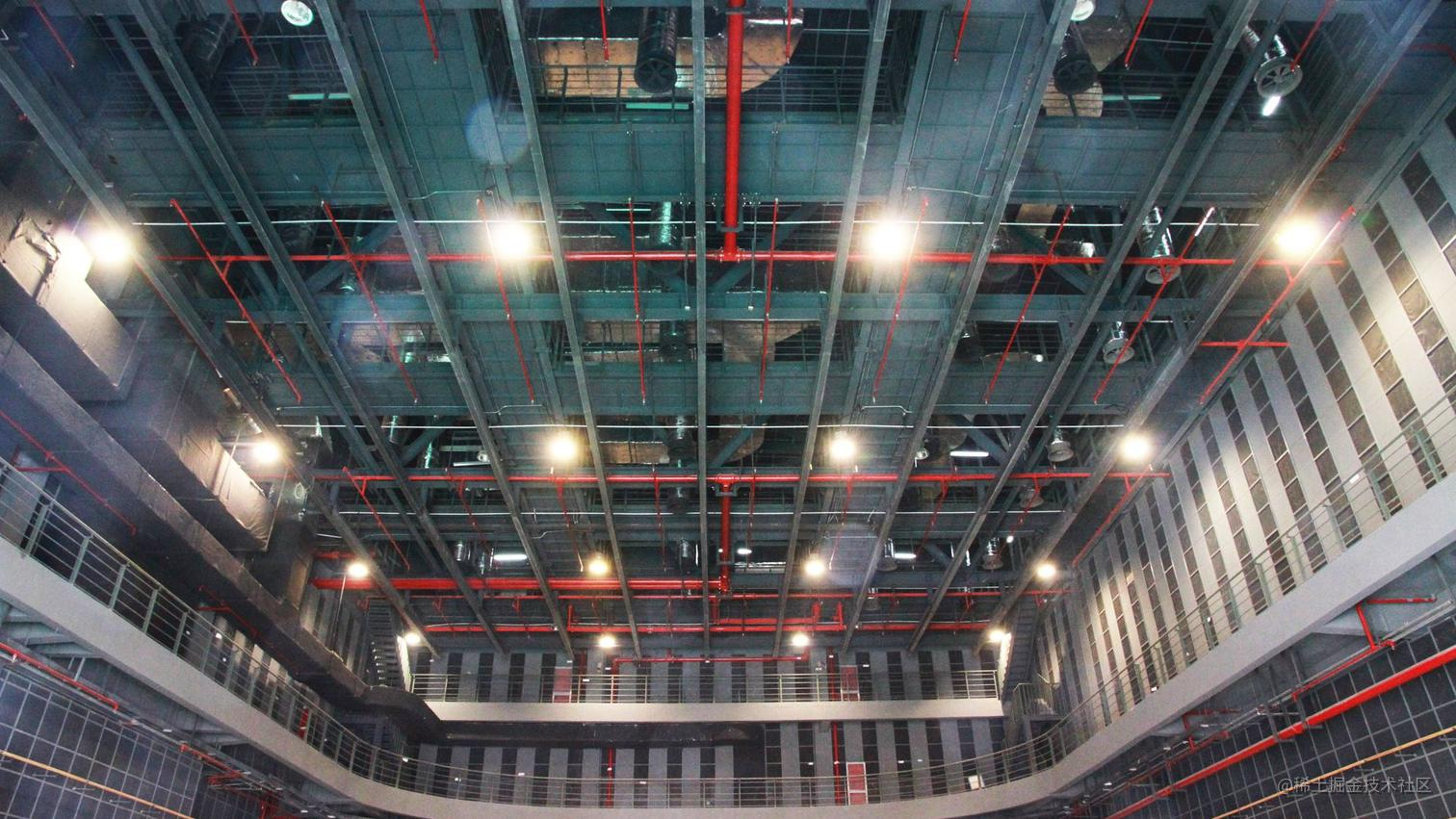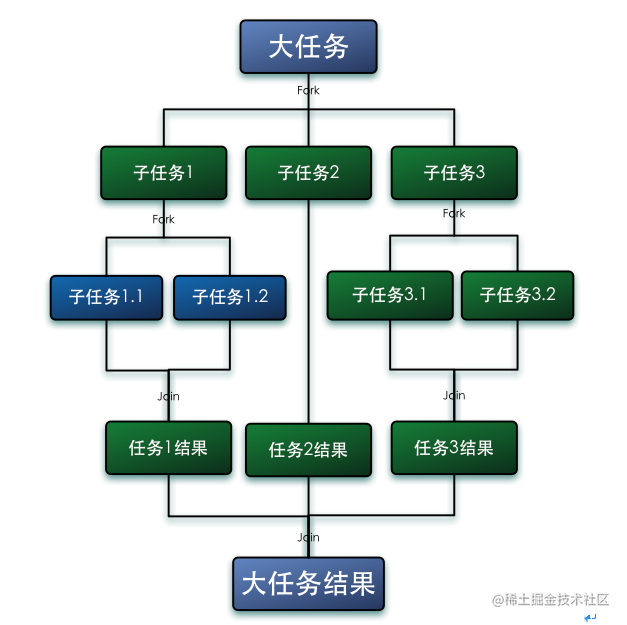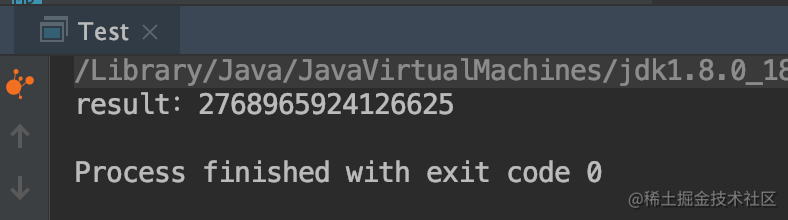# 如何高效的使用并行流#### Fork/Join框架

Fork/Join框架采用的是思想就是分而治之，把大的任务拆分成小的任务，然后放入到独立的线程中去计算，同时为了最大限度的利用多核CPU，采用了一个种`工作窃取`的算法来运行任务，也就是说当某个线程处理完自己工作队列中的任务后，尝试当其他线程的工作队列中窃取一个任务来执行，直到所有任务处理完毕。所以为了减少线程之间的竞争，通常会使用双端队列，被窃取任务线程永远从双端队列的头部拿任务执行，而窃取任务的线程永远从双端队列的尾部拿任务执行；在百度找了一张图• 使用`RecursiveTask` 使用Fork/Join框架首先需要创建自己的任务，需要继承`RecursiveTask`，实现抽象方法
``````protected abstract V compute();

``````if(任务已经不可拆分){
return 顺序计算结果;
} else {
1.任务拆分成两个子任务
2.递归调用本方法，拆分子任务
3.等待子任务执行完成
4.合并子任务的结果
}

• Fork/Join实战

``````long result = LongStream.rangeClosed(1, 100000000)
.reduce(0, Long::sum);
System.out.println("result：" + result);

``````public class SumRecursiveTask extends RecursiveTask<Long> {
private long[] numbers;
private int start;
private int end;

this.numbers = numbers;
this.start = 0;
this.end = numbers.length;
}

public SumRecursiveTask(long[] numbers, int start, int end) {
this.numbers = numbers;
this.start = start;
this.end = end;
}

@Override
protected Long compute() {
int length = end - start;
if (length < 20000) {  //小于20000个就不在进行拆分
return sum();
}
}

private long sum() {
int sum = 0;
for (int i = start; i < end; i++) {
sum += numbers[i];
}
return sum;
}

public static void main(String[] args) {
long[] numbers = LongStream.rangeClosed(1, 100000000).toArray();

Long result = new ForkJoinPool().invoke(new SumRecursiveTask(numbers));
System.out.println("result：" +result);
}
}

Fork/Join默认的线程数量就是你的处理器数量，这个值是由`Runtime.getRuntime().available- Processors()`得到的。 但是你可以通过系统属性`java.util.concurrent.ForkJoinPool.common. parallelism`来改变线程池大小，如下所示： `System.setProperty("java.util.concurrent.ForkJoinPool.common.parallelism","12");` 这是一个全局设置，因此它将影响代码中所有的并行流。目前还无法专为某个 并行流指定这个值。因为会影响到所有的并行流，所以在任务中经历避免网络/IO操作，否则可能会拖慢其他并行流的运行速度

#### parallelStream

• 常见的使用方式 1.串行流转化成并行流
``````LongStream.rangeClosed(1,1000)
.parallel()
.forEach(System.out::println);

2.直接生成并行流

`````` List<Integer> values = new ArrayList<>();
for (int i = 0; i < 10000; i++) {
}
values.parallelStream()
.forEach(System.out::println);

• 正确的使用parallelStream

``````public static void main(String[] args) {
Summer summer = new Summer();
LongStream.rangeClosed(1, 100000000)
.parallel()
System.out.println("result：" + summer.sum);

}

static class Summer {
public long sum = 0;

sum += value;
}
}``````long result = LongStream.rangeClosed(1, 100000000)
.parallel()
.reduce(0, Long::sum);
System.out.println("result：" + result);

`Spliterator`是Java8加入的新接口，是为了并行执行任务而设计的。

``````public interface Spliterator<T> {

Spliterator<T> trySplit();

long estimateSize();

int characteristics();
}

trySplit: 对所有的元素进行拆分成小的子部分，如果已经不能拆分就返回null

estimateSize: 当前拆分里面还剩余多少个元素

characteristics: 返回当前Spliterator特性集的编码

#### 总结

1. 要证明并行处理比顺序处理效率高，只能通过测试，不能靠猜测（本文累加的例子在多台电脑上运行了多次，也并不能证明采用并行来处理累加就一定比串行的快多少，所以只能通过多测试，环境不同可能结果就会不同）
2. 数据量较少，并且计算逻辑简单，通常不建议使用并行流
3. 需要考虑流的操作时间消耗
4. 在有些情况下需要自己去实现拆分的逻辑，并行流才能高效